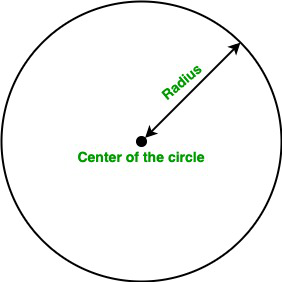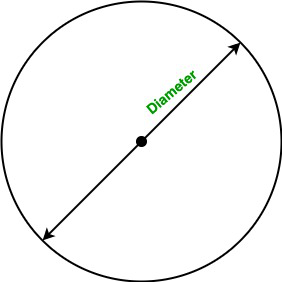A circle is a simple shape consisting of all the points in the plane that are equidistant from a point known as the center of the circle. In this article, we will learn how to find the area of the circle using the method overloading.

Terminology:

• Method Overloading: Method overloading allows different methods to have the same name, but different signatures where the signature can differ by the number of input parameters or type of input parameters or both.
• Area: A quantity that represent the extent of a 2-dimensional figure or shape in the plane is known as an area.
• Radius: The line segment from the center to any point of the circle is known as radius.
• Diameter: The line segment whose endpoints lie on the circle and passed through the center is known as the diameter of the circle. It is also known as the largest distance between any two points on the circle.

#### Area of the Circle

The area of the circle is the product of the square of the radius of the circle and the value of PI. We can simply calculate the area of the circle using the following formula:

• Using the radius of the circle:Formula:

Area of the circle:  A = π * r2

Here, r is the radius of the circle.

• Using the diameter of the circle:Formula:

Area of the circle:  A = (π / 4) * d2

Here, r is the radius of the circle.

Note: The value of PI in java is 3.141592653589793.

Below is the implementation of the above approach:

Example 1:

## Java

 `// Java program to find the area of` `// the circle using Method Overloading`   `import` `java.io.*;`   `class` `Circle {`   `    ``static` `final` `double` `PI = Math.PI;`   `    ``// Overloaded Area() function to` `    ``// calculate the area of the circle.` `    ``// It takes one double parameter` `    ``void` `Area(``double` `r)` `    ``{` `        ``double` `A = PI * r * r;`   `        ``System.out.println(``"Area of the circle is :"` `+ A);` `    ``}`   `    ``// Overloaded Area() function to` `    ``// calculate the area of the circle.` `    ``// It takes one float parameter` `    ``void` `Area(``float` `r)` `    ``{` `        ``double` `A = PI * r * r;`   `        ``System.out.println(``"Area of the circle is :"` `+ A);` `    ``}` `}`   `class` `GFG {`   `    ``// Driver code` `    ``public` `static` `void` `main(String[] args)` `    ``{`   `        ``// Creating object of Circle class` `        ``Circle obj = ``new` `Circle();`   `        ``// Calling function` `        ``obj.Area(``5``);` `        ``obj.Area(``2.5``);` `    ``}` `}`

Output

```Area of the circle is :78.53981633974483
Area of the circle is :19.634954084936208```

Time Complexity: O(1)

Auxiliary Space: O(1)

Example 2:

## Java

 `// Java program to find the area of` `// the circle when the diameter is given` `// using Method Overloading`   `import` `java.io.*;`   `class` `Circle {`   `    ``static` `final` `double` `PI = Math.PI;`   `    ``// Overloaded Area() function to` `    ``// calculate the area of the circle.` `    ``// It takes one double parameter` `    ``void` `Area(``double` `D)` `    ``{` `        ``double` `A = (PI / ``4``) * D * D;`   `        ``System.out.println(``"Area of the circle is :"` `+ A);` `    ``}`   `    ``// Overloaded Area() function to` `    ``// calculate the area of the circle.` `    ``// It takes one float parameter` `    ``void` `Area(``float` `D)` `    ``{` `        ``double` `A = (PI / ``4``) * D * D;`   `        ``System.out.println(``"Area of the circle is :"` `+ A);` `    ``}` `}`   `class` `GFG {`   `    ``// Driver code` `    ``public` `static` `void` `main(String[] args)` `    ``{`   `        ``// Creating object of Circle class` `        ``Circle obj = ``new` `Circle();`   `        ``// Calling function` `        ``obj.Area(``10``);` `        ``obj.Area(``20.4``);` `    ``}` `}`

Output

```Area of the circle is :78.53981633974483
Area of the circle is :326.851299679482```

Time complexity: O(1) since performing constant operations

Auxiliary Space: O(1)

Whether you're preparing for your first job interview or aiming to upskill in this ever-evolving tech landscape, GeeksforGeeks Courses are your key to success. We provide top-quality content at affordable prices, all geared towards accelerating your growth in a time-bound manner. Join the millions we've already empowered, and we're here to do the same for you. Don't miss out - check it out now!